# Hydraulic Structures for Discharge Measurement Flow Measurement Measurement

• Slides: 19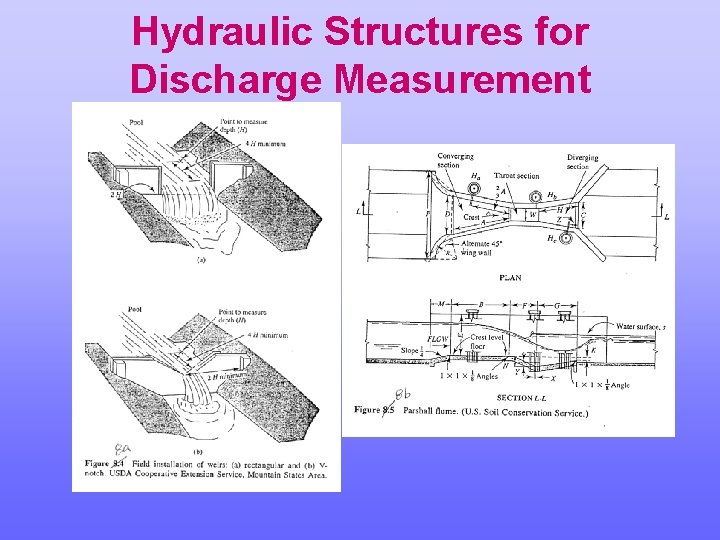Hydraulic Structures for Discharge Measurement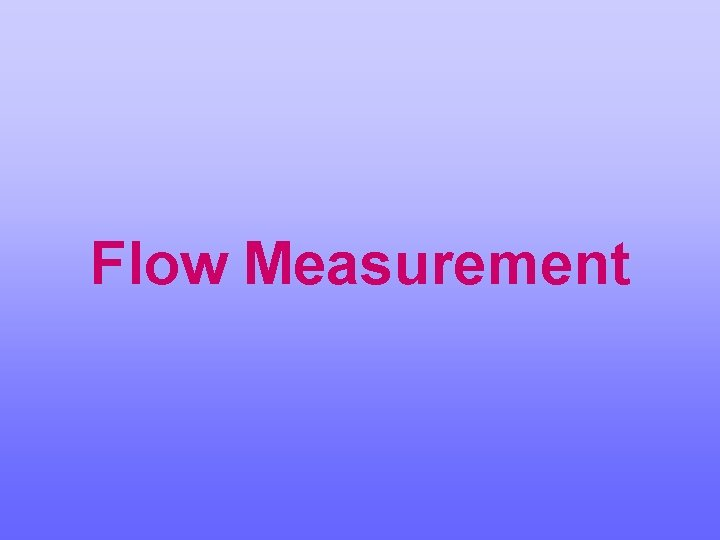Flow MeasurementMeasurement Approaches • Direct methods (stream gaging) – Current-meter method – Floats – Tracer-dilution technique – Ultrasonic method – Electromagnetic methodMeasurement Approaches • Hydraulic Devices – Weirs and notches – Orifices – Flumes • Indirect Techniques – Slope-area method – Rating CurveDefinition of Terms • Stage: Height of water surface above a fixed datum • Rating: Relationship between stream stage and discharge • Control: Feature that results in a one-to-one relationship between stage and discharge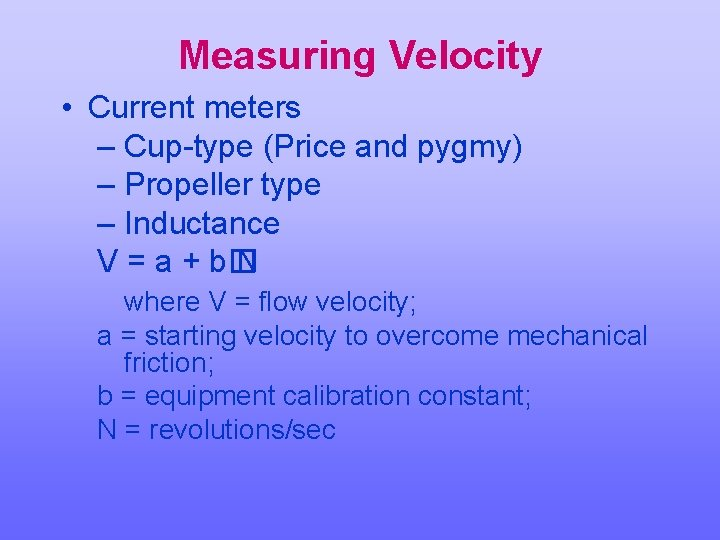Measuring Velocity • Current meters – Cup-type (Price and pygmy) – Propeller type – Inductance V = a + b� N where V = flow velocity; a = starting velocity to overcome mechanical friction; b = equipment calibration constant; N = revolutions/sec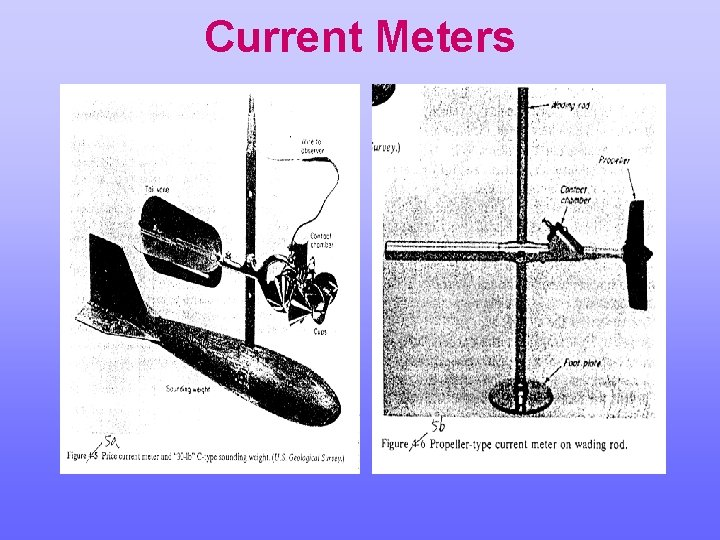Current Meters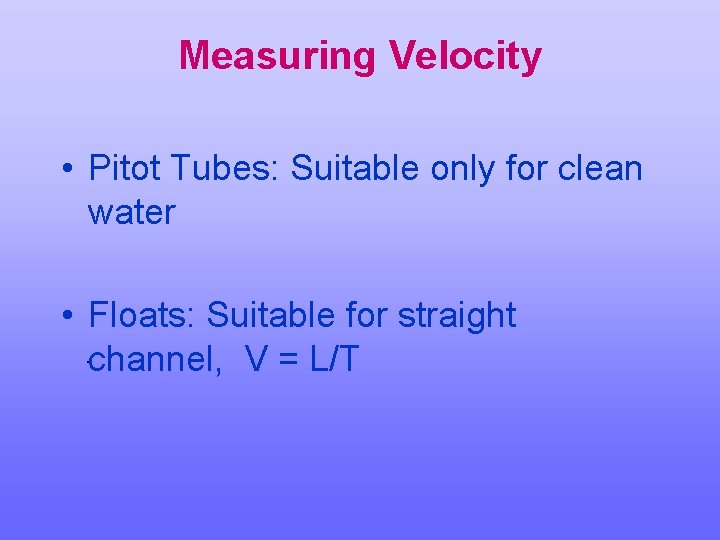Measuring Velocity • Pitot Tubes: Suitable only for clean water • Floats: Suitable for straight. channel, V = L/TVelocity Distributions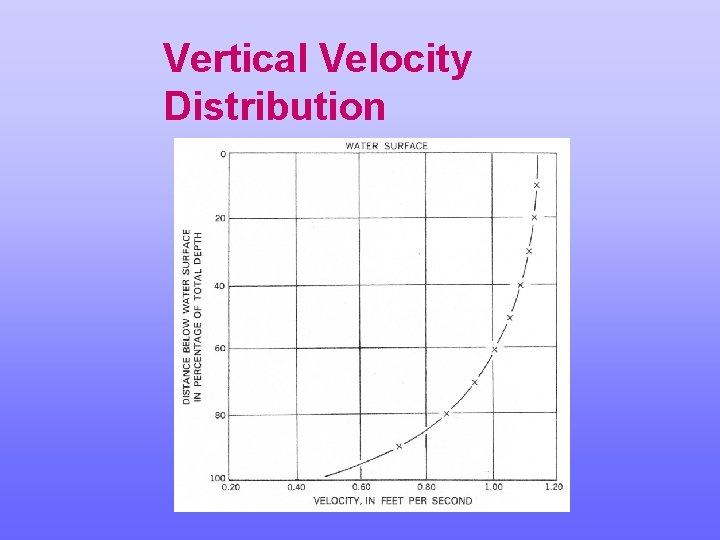Vertical Velocity Distribution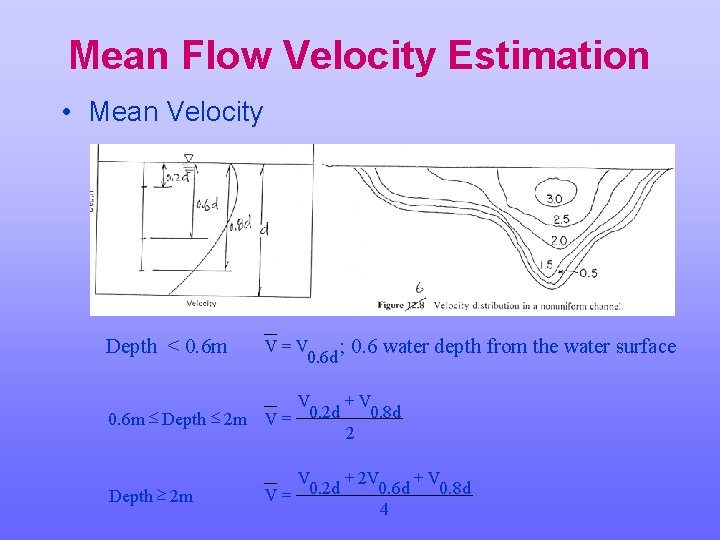Mean Flow Velocity Estimation • Mean Velocity Depth < 0. 6 m V=V ; 0. 6 water depth from the water surface 0. 6 d +V V 0. 2 d 0. 8 d 0. 6 m £ Depth £ 2 m V = 2 Depth ³ 2 m + 2 V +V V 0. 2 d 0. 6 d 0. 8 d V= 4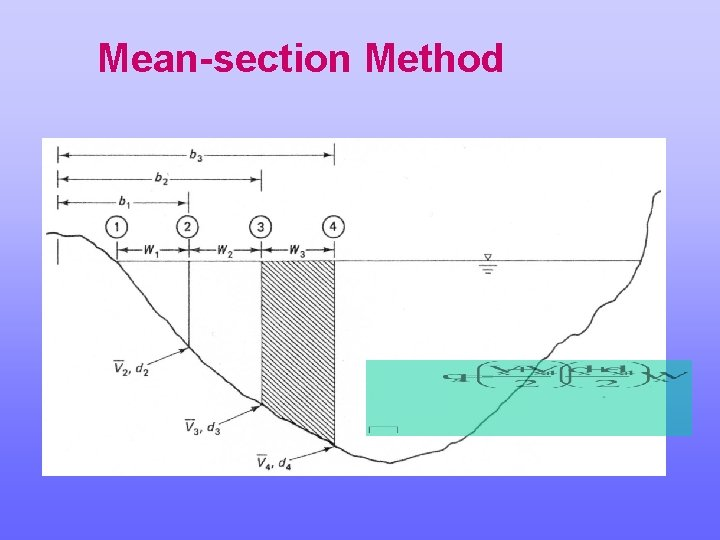Mean-section Method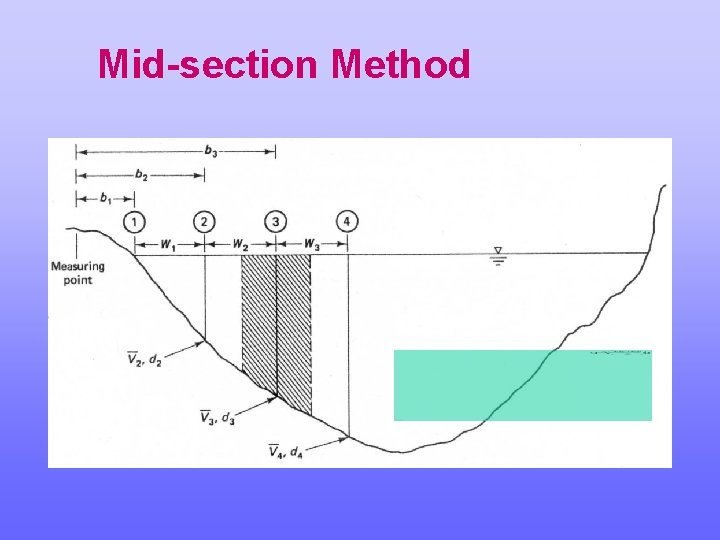Mid-section MethodStage-Discharge Ratings • If it is not possible to install a weir or flume (large channel), need to develop a stage-discharge rating • 3 basic types – Simple stage-discharge • Control produces one-to-one rating – Slope-stage-discharge – Velocity-stage-discharge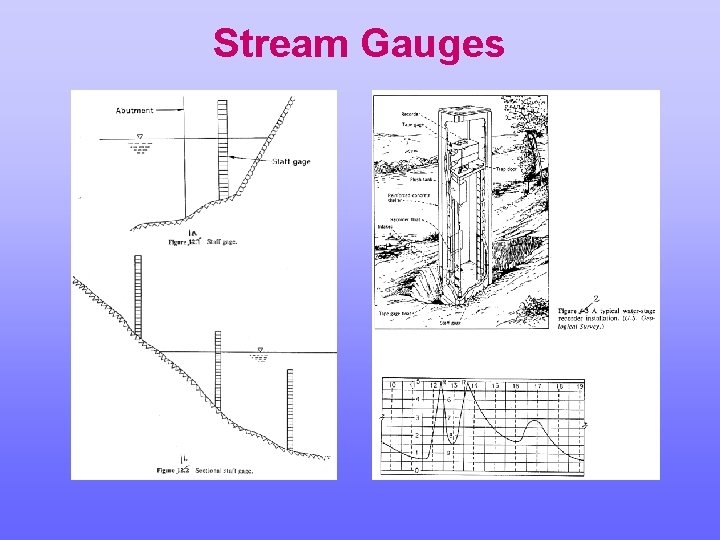Stream Gauges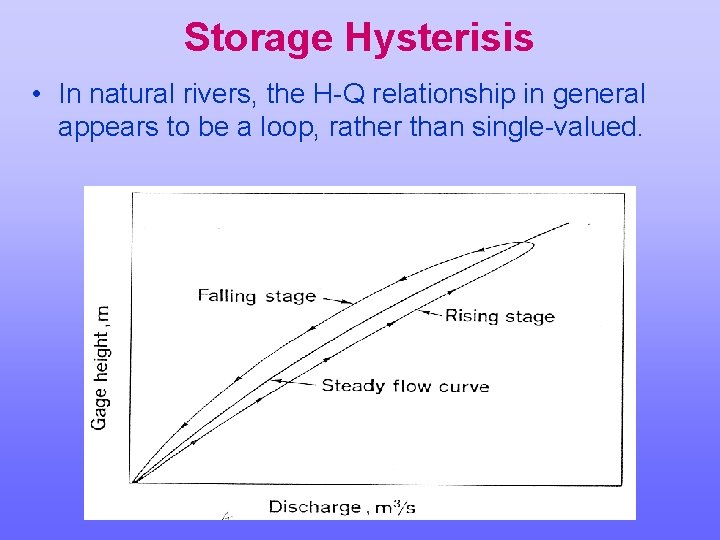Storage Hysterisis • In natural rivers, the H-Q relationship in general appears to be a loop, rather than single-valued.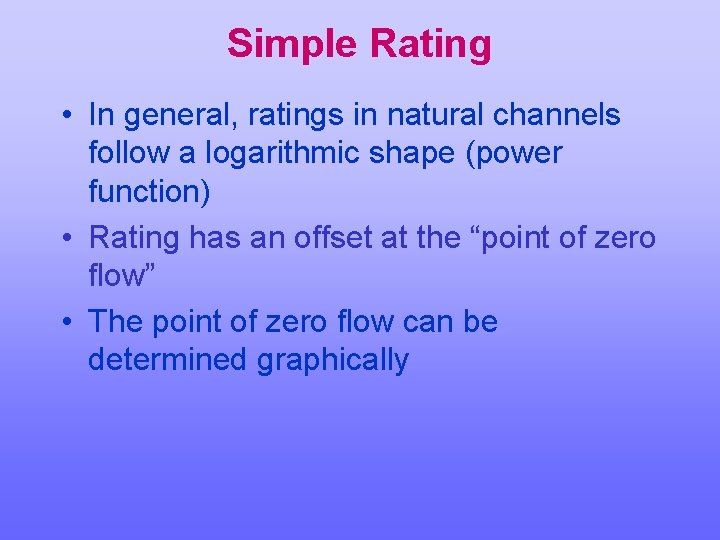Simple Rating • In general, ratings in natural channels follow a logarithmic shape (power function) • Rating has an offset at the “point of zero flow” • The point of zero flow can be determined graphicallyUse of Manning Equation • Stage is also related to the outflow via a relationship such as Manning's equation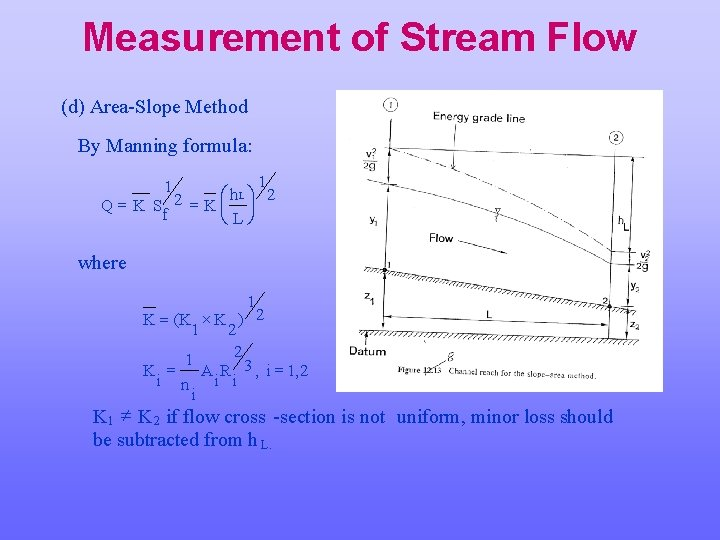Measurement of Stream Flow (d) Area-Slope Method By Manning formula: 1 Lö 2 æ h Q = K S 2 = Kç ÷ f èLø 1 where 1 K = (K ´ K ) 2 1 2 2 1 K = A R 3 , i = 1, 2 i n i i i K 1 ¹ K 2 if flow cross -section is not uniform, minor loss should be subtracted from h L.